Identify worst cell relative to other cells in serially connected lithium-ion battery pack

Since R2023a

## Syntax

``adjacentPairCorrelationModel(data)``
``adjacentPairCorrelationModel(data,Name=Value)``
``[worstcell,corrcf] = adjacentPairCorrelationModel(___)``

## Description

example

````adjacentPairCorrelationModel(data)` analyzes the battery data in `data` to determine the voltage correlation coefficients between adjacent cells in a serially connected lithium-ion battery pack, and plots the results.The lower the correlation coefficient is between a pair of adjacent cells, the more likely it is that one of the cells in the pair has a fault condition, such as an internal short circuit . The plot legend indicates the cell pair with the lowest correlation coefficients. Using this syntax also displays the index of the worst cell in the pack.The algorithm imposes no restrictions on battery activity during the collection of `data`. The battery can be in any operating sequence, including charging, discharging, and standby phases.```

example

````adjacentPairCorrelationModel(data,Name=Value)` incorporates additional options specified by one or more name-value arguments. For example, increase the `SquarewaveAmp` argument to a value greater than the default value of 0.003 to accommodate higher levels of noise when the batteries are at rest.```

example

````[worstcell,corrcf] = adjacentPairCorrelationModel(___)` returns the index of the worst cell `worstcell` and the estimated correlation coefficients between each adjacent pair. You can use this syntax with any of the previous input-argument combinations.```

## Examples

collapse all

Load the data, which represents an operating profile for an eight-cell battery pack in which one cell is experiencing an internal short circuit. The profile includes standby, driving, charging, and balancing phases. The data consists of 10 columns that contain the sample time (column 1), battery voltages (columns 2–9), and pack current (column 10).

`load internalShortCircuit.mat internalShortCircuit`

For the adjacent-pair correlation model, you need only the cell voltages. Extract the voltage columns into the data set `data`.

`data = internalShortCircuit(:,2:end-1);`

Plot the battery voltages.

```plot(data) legend('Voltages (V)') title('InternalShortCircuit Dataset - Voltages') ylabel('Voltage (V) Values')```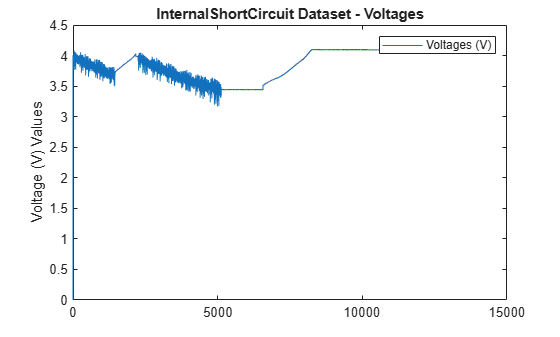The voltages appear to track closely, but at this scale it is hard to differentiate them.

Zoom into the region after t = 4000 to show the individual voltages more clearly.

```xlim([4000 4010]) ylim([3.4 3.6]) title('Voltage Separation') ylabel('Voltages (V)')```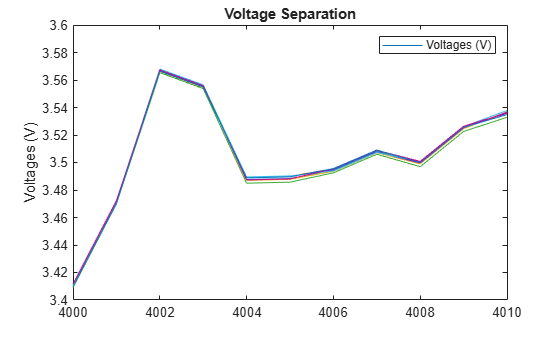The voltages track closely together. There is no cell that is obviously degrading relative to the others.

Identify the worst cell using `adjacentPairCorrelationModel`.

`adjacentPairCorrelationModel(data)`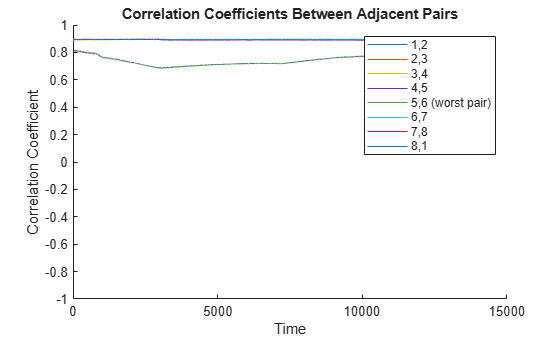```ans = 5 ```

The correlation model identifies cell pair 5,6 as the worst pair and cell 5 as the worst cell.

Load the data, which is contained in a table, and show the first two rows.

```load internalShortCircuitTbl.mat internalShortCircuitTbl head(internalShortCircuitTbl,2)```
``` Time Cell1 Cell2 Cell3 Cell4 Cell5 Cell6 Cell7 Cell8 Current ____ ______ ______ _____ ______ ______ ______ ______ _____ _______ 0 0 0 0 0 0 0 0 0 0 5 4.0037 4.0018 4.002 4.0012 4.0029 4.0012 4.0042 4.002 0 ```

The adjacent-pair correlation model does not require the `Current` values. Extract the time and voltage values into the timetable `data`.

```data = internalShortCircuitTbl(:,1:end-1); head(data,2)```
``` Time Cell1 Cell2 Cell3 Cell4 Cell5 Cell6 Cell7 Cell8 ____ ______ ______ _____ ______ ______ ______ ______ _____ 0 0 0 0 0 0 0 0 0 5 4.0037 4.0018 4.002 4.0012 4.0029 4.0012 4.0042 4.002 ```

Use `adjacentPairCorrelationModel` to identify the worst cell. Use `Time` to specify the time variable.

`adjacentPairCorrelationModel(data(:,2:end),Time=data.Time)`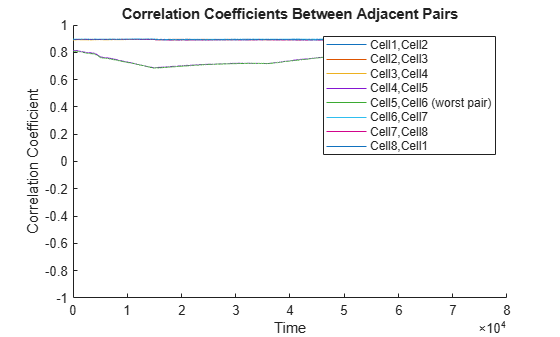```ans = 5 ```

The function identifies the worst pair and the worst cell within the pair.

Load the data, which is contained in a timetable, and extract the cell voltages into `data`.

```load internalShortCircuitTT.mat internalShortCircuitTT data = internalShortCircuitTT(:,1:end-1);```

Run `adjacentPairCorrelationModel` using output arguments to store the analysis results.

`[worstcell,corrcoef] = adjacentPairCorrelationModel(data);`

Identify the worst cell.

`iwc = worstcell`
```iwc = 5 ```

Plot the estimated correlation coefficient curves. For the legend, use the `VariableNames` property of `data` to access the cell names. With this plotting approach, the curve for each cell in the legend represents the correlation coefficient curve between that cell and the cell to the right in `data`.

For example, `Cell1` represents the correlation curve between `Cell1` and `Cell2`. `Cell8` represents the correlation curve between `Cell8` and `Cell1`.

```plot(corrcoef.Time,corrcoef.Variables) title('Correlation Coefficient Curves') legend(corrcoef.Properties.VariableNames) ylabel('corrcoef')```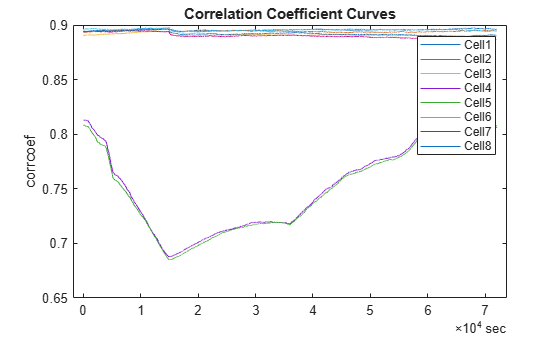Cell 5, which the function identifies as the worst cell, has the lowest pair of correlation coefficient curves.

## Input Arguments

collapse all

Battery cell voltage, specified as a `table`, a `timetable`, or a matrix that contains ns rows, where ns is the number of samples, and nc columns or variables, where nc is the number of cells.

If your measurement data also includes an explicit column for time, you must exclude that column from the `data` argument when you call `adjacentPairCorrelationModel`. However, you can access the time data for plotting by using the `Time` name-value argument.

If `data` is in the form of a timetable, you can use `data` directly with `adjacentPairCorrelationModel`. Use timetable properties such as `data.Time` or `data.Variables` to extract information for additional analysis or plotting.

Example: `adjacentPairCorrelationModel(data(:,2:end),Time=data(:,1))` analyzes the cell voltage correlations using the voltage data in the matrix or table `data`, and plots the results against the time data in the first column.

### Name-Value Arguments

Specify optional pairs of arguments as `Name1=Value1,...,NameN=ValueN`, where `Name` is the argument name and `Value` is the corresponding value. Name-value arguments must appear after other arguments, but the order of the pairs does not matter.

Example: `adjacentPairCorrelationModel(data,WindowSize=size(data,1)/2)` sets the window size to half the number of samples when the number of samples is even.

Time data to use for plotting, specified as numeric vector, a `duration` vector, or a `datetime` vector of length ns, where ns is the number of samples in `data`. `'Time'` can be a column in the dataset used for `data` or a separate vector.

If `data` is a `timetable`, the time information in `data` takes precedence and the software ignores the `'Time'` specification.

If `data` is a table or a matrix and `'Time'` is not specified, then the function plots the results against the index vector [1:ns].

Example: `adjacentPairCorrelationModel(data,Time=tv)` specifies the time vector `tv`, which has the same number of rows as `data` but is separate from `data`.

Length of the moving window for computing the correlation coefficient, specified as a positive integer scalar. The default value is equal to the number of rows, or samples, in `data`.

• When the window size is odd, each moving window is centered on the current element in `data`. For instance, if the window size is `49`, the window is centered on the 25th element within the window.

• When the window size is even, each window is centered about the current and previous elements.

• When there are not enough points remaining at the end to fill the window, the software truncates the window size so that the final window is full.

Setting the window size is a balance between sensitivity to faults and insensitivity to noise. When `WindowSize` is small, the correlation coefficients are more sensitive to noises and their impact on voltage changes, which can result in false fault detections. Conversely, when `WindowSize` is large, the changes in voltage are less detectable and might be missed.

Example: `adjacentPairCorrelationModel(data,WindowSize=size(data,1)/2)` sets the window size to half the number of samples when the number of samples is even.

Amplitude of the square wave that is added to `data`, specified as a non-negative numeric scalar or, to disable the square wave, 0.

When the battery is at rest, noise dominates the correlation coefficient calculations and the coefficients for healthy pairs are close to zero, interfering with the identification of the true worst cell. Adding the square wave raises the coefficient values above the noise floor. A good square wave amplitude is three times the noise level in `data`. With this amplitude, the correlation coefficients of healthy pairs are around 0.9, allowing truly faulty cells with lower correlation coefficients to be detected correctly.

Example: `adjacentPairCorrelationModel(data,SquarewaveAmp=0.1)` sets the square wave amplitude to 0.1.

## Output Arguments

collapse all

Index of the worst cell, returned as an integer that corresponds to the cell that has the lowest `corrcf` curves with adjacent cells. That is, the worst cell is the cell that deviates the most from its adjacent neighbors.

If the two minimum `corrcf` curves do not share the same cell, then the software determines the worst cell as follows:

• Identify the adjacent pair with the lowest `corrcf` curve as the pair that must contain the worst cell.

• Within this pair, compare the raw voltage of the two cells. Lower voltage is an indication of degradation. Identify the cell with the lower raw voltage as the worst cell.

For example, suppose that the cell pair 4,5 has the lowest `corrcf` curve, but cell pairs 3,4 and 5,6 both have `corrcf` curves that are higher than the second lowest adjacent-pair `corrcf` curve. If the raw voltage of cell 4 is less than the raw voltage of cell 5, then the software returns cell 4 as the worst cell.

Correlation coefficients between each adjacent column pair in `data`, returned as a table, timetable, or matrix. The data type of `corrcf` is consistent with the data type of `data`.

For example, `corrcf(:,1)` represents the correlation coefficient curve between cells 1 and 2. For an eight-cell battery pack, `corrcf(:,8)` represents the correlation coefficient curve between cells 8 and 1.

 Xia, Bing, Yunlong Shang, Truong Nguyen, and Chris Mi. “A Correlation Based Fault Detection Method for Short Circuits in Battery Packs.” Journal of Power Sources 337 (January 2017): 1–10. https://doi.org/10.1016/j.jpowsour.2016.11.007.